In this page we have Linked Type Comprehension questions on rotation for JEE Main/Advanced . Hope you like them and do not forget to like , social share and comment at the end of the page.
A particle of mass m moves such a way that momentum vector varies as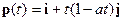Where i and j are unit vector across x and y axis
Question 1 Find the Force vector on the mass where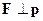a) j
b) –j
c) i +j
d) i -j
Question 2 Find the impulse on the mass over the period t and t+?t
a)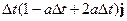b)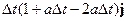c)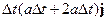d)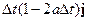An object of mass M collides with a frictionless surface. The surface is assumed to be x-axis and object is coming at an angle θ to the x-axis. The object bounces from the surface but the collision is not elastic
The initial velocity vector of the object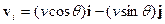The vertical component of the velocity undergoes a change due to the impact. The magnitude is fraction e of the original vertical components
The situation is depicted below in the figure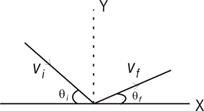Question 3 Find the velocity vector after the collision
a)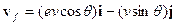b)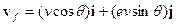c)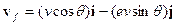d)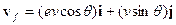Question 4 Find the final angle made with the horizontal
a)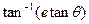b)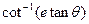c)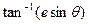d)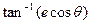Question 5 Find the change in momentum ?p due to the impact
a)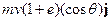b)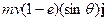c)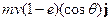d)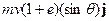Question 6 Which one of the following is true about the case?
a) Momentum remain conserved in the X –direction
b) There is no loss of kinetic energy of the object
c) The force during the collision acts in upward direction on the object
d) The ratio of the final KE to the initial KE is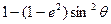Multiple Choice Questions
Question 7: An object of mass M is at the origin and is at rest. Suddenly an explosion happens in the object and object is divided three equal parts.
The first part goes with the velocity (v0i+v0 j) .
The second part goes with the velocity v0 j
What is the velocity vector for the third part?
a) (v0i+v0 j)
b) (-v0i-2v0 j)
c) (v0i-v0 j)
d) None of these
Question 8: A flat car filled with sand is moving with velocity v. The flat car is leaking sand at the rate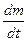. Find the velocity of the flat car after ½ amount has leaked
a) v
b) v/2
c) v/4
d)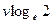A rocket ascends from rest in a uniform gravitational field by ejecting exhaust with constant speed u. The rate of expulsion is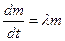where m is the instantaneous mass of the rocket and λ is constant. The rocket is also retarded by air resistance with a force (mbv) where b is constant
Question 9 Find the velocity of the rocket as a function of time
a)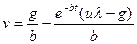b)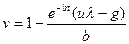c)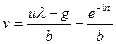d)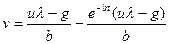Question 10 Find the terminal velocity of the rocket
a)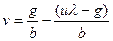b)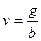c)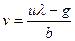d)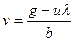Solutions